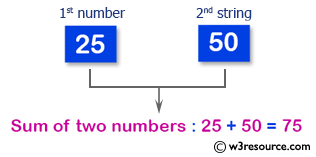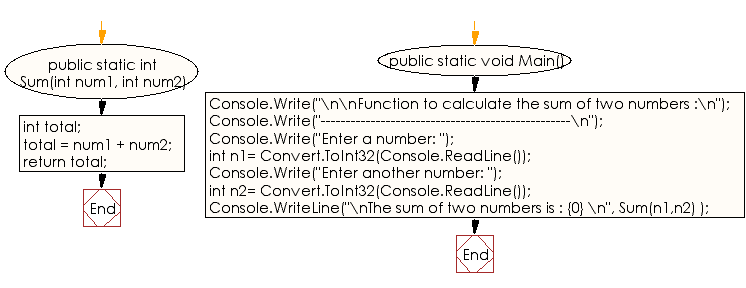﻿ C# Sharp Exercises: Function to calculate the sum of two numbers - w3resource# C# Sharp Exercises: Function: Function to calculate the sum of two numbers

## C# Sharp Function: Exercise-3 with Solution

Write a program in C# Sharp to create a function for the sum of two numbers.

Pictorial Presentation:Sample Solution:

C# Sharp Code:

``````using System;
public class funcexer3
{
public static int Sum(int num1, int num2)
{
int total;
total = num1 + num2;
return total;
}

public static void Main()
{
Console.Write("\n\nFunction to calculate the sum of two numbers :\n");
Console.Write("--------------------------------------------------\n");
Console.Write("Enter a number: ");
int n1= Convert.ToInt32(Console.ReadLine());
Console.Write("Enter another number: ");
int n2= Convert.ToInt32(Console.ReadLine());
Console.WriteLine("\nThe sum of two numbers is : {0} \n", Sum(n1,n2) );
}
}
```
```

Sample Output:

```Function to calculate the sum of two numbers :
--------------------------------------------------
Enter a number: 25
Enter another number: 50

The sum of two numbers is : 75
```

Flowchart :C# Sharp Code Editor:

Improve this sample solution and post your code through Disqus

What is the difficulty level of this exercise?

﻿